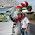### Division of fractions conceptually

I've made two videos on a very important topic (I feel) of fraction division.

The two videos show a step-by-step approach for teaching division of fractions conceptually:

1. Start with sharing divisions that divide evenly. For example, 4/7 ÷ 2 can be thought of as "Two people share 4/7 of a pie evenly. How much of the pie does each person get?"

Children can figure these out mentally without using any rule.

2. Continue to measurement divisions where we think, "How many times does the divisor fit into the dividend?". Again, the problems should first be designed so that the divisions are even. For example, 4 ÷ (1/2) means "How many times does 1/2 fit into 4?" Or, 3 1/5 ÷ 2/5 means "How many times does 2/5 fit into 3 1/5?" Again, no rule is necessary to solve these - just logical thinking.

3. Next, study measurement divisions with the dividend of one. This leads to the concept of reciprocal numbers. For example, 1 ÷ 3/4 is thought of as "How many times does 3/4 fit into 1?" As I show in the video, and you should show visually, 3/4 fits into 3/4 once, and into the leftover piece of 1/4 it fits 1/3 of the way. So, all total 3/4 fits into 1 exactly 1 1/3 times.

Students should do a sufficient number of these kinds of problems so that they get familiar with the thinking process. After that, take some of the problems they have solved, and write the answers as fractions instead of mixed numbers. For example we found above that 1 ÷ 3/4 = 1 1/3. Write 1 1/3 as a fraction, and you get 1 ÷ 3/4 = 4/3. Now, 3/4 and 4/3 are reciprocal numbers. If you multiply them, you get 1. And this happens with every such division problem.

4. Lastly, we apply this neat pattern with reciprocal numbers to arbitrary fraction division problems, and thus arrive at the shortcut for fraction division.

For example, 7 ÷ (4/5). Use the helping problem of 1 ÷ (4/5) = 5/4. Since 4/5 fits into 1 exactly 5/4 times, it fits into 7 exactly seven times that many times, or 7 × 5/4 = 8 3/4 times. Similarly, let's consider 6/7 ÷ (3/4). First look at the helping problem 1 ÷ (3/4) = 4/3. Since 3/4 fits into 1 exactly 4/3 times, it fits into 6/7 exactly 6/7 that many times. The answer is therefore 6/7 × 4/3 = 24/21 = 1 1/7.

We can notice that for any fraction division problem, we end up multiplying the dividend by the reciprocal of the divisor! Students can actually notice and understand this shortcut on their own, using this method. It is no longer just a rule handed to them on a platter as, "Swallow this, no questions." It becomes a concept they can understand.

### CommentsThis is the first time I have been to your blog and seen your videos. Great job, thank you, the concept was very clear to see and understand. I used this with my son and showed him the "tricks" and he finally had some success with this. Thank you again, your work is appreciated!!!

Sheila